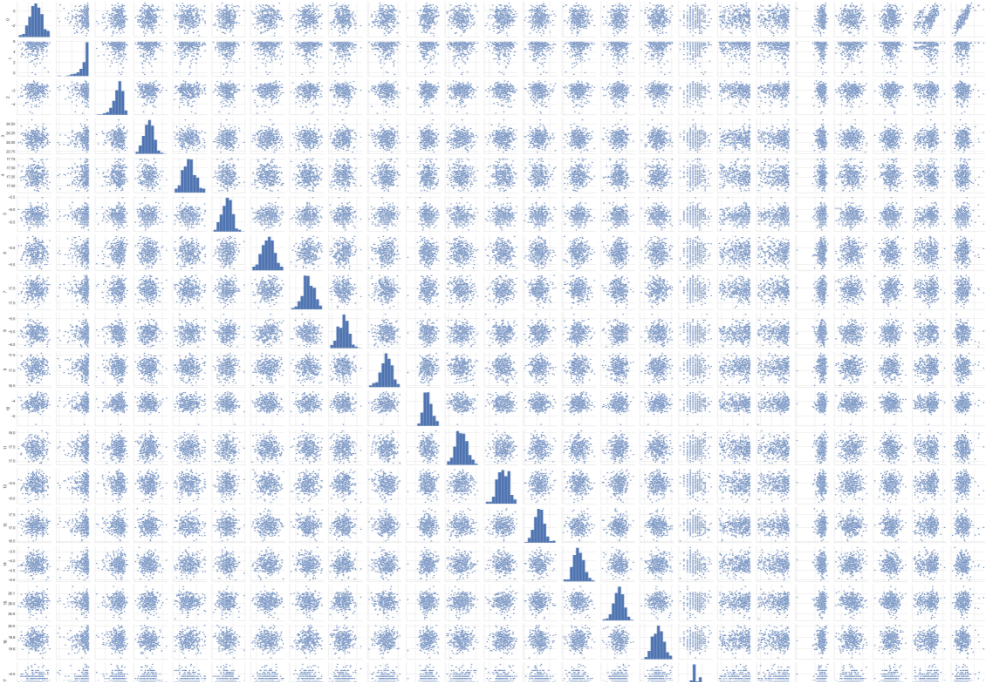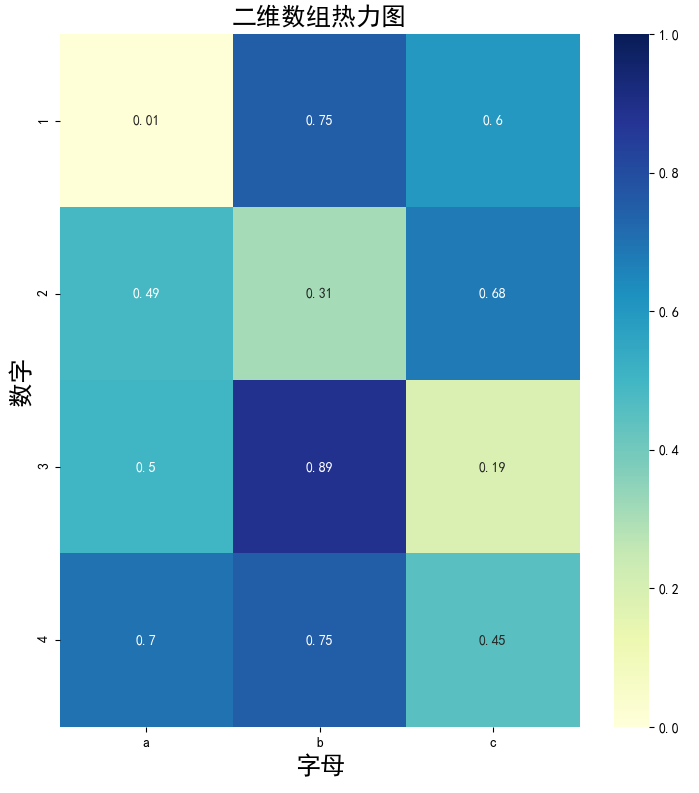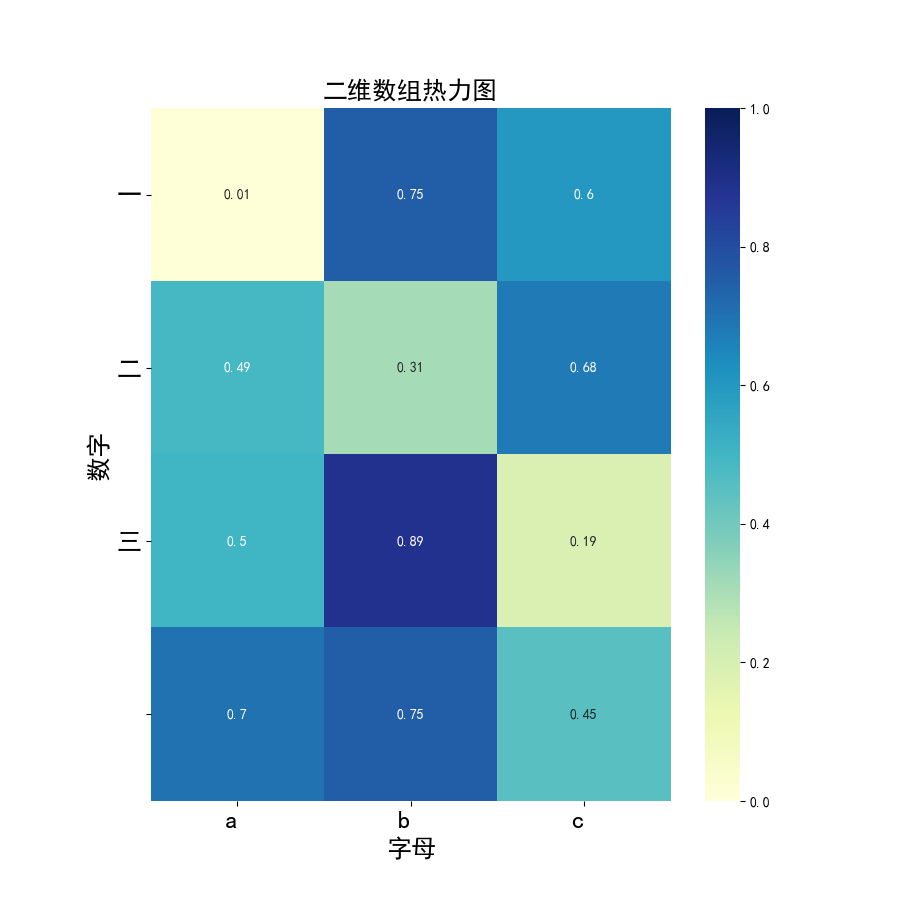• ## python画混淆矩阵图

千次阅读 2020-10-18 16:33:27
1. 计算混淆矩阵 from sklearn.metrics import confusion_matrix # 导入计算混淆矩阵的包 ...2. 用seaborn的热力图画混淆矩阵图 import seaborn as sns #导入包 xtick=['1','2','3','4','5','6','7'
1. 计算混淆矩阵
from sklearn.metrics import confusion_matrix # 导入计算混淆矩阵的包

C1= confusion_matrix(True_label, T_predict1) #True_label 真实标签 shape=(n,1);T_predict1 预测标签 shape=(n,1)2. 用seaborn的热力图画混淆矩阵图
import seaborn as sns #导入包
xtick=['1','2','3','4','5','6','7','8','9','10']
ytick=['1','2','3','4','5','6','7','8','9','10']

sns.heatmap(C1,fmt='g', cmap=name,annot=True,cbar=False,xticklabels=xtick, yticklabels=ytick) #画热力图,annot=True 代表 在图上显示 对应的值， fmt 属性 代表输出值的格式，cbar=False, 不显示 热力棒
plt.show()展开全文• 矩阵图法就是从多维问题的事件中，找出成对的因素，排列成矩阵图，然后根据矩阵图来分析问题，确定关键点的方法。它是一种通过多因素综合思考，探索问题的好方法。从问题事项中找出成对的因素群，分别排列成行和列，...


目录
1 矩阵图法简介2 代码3 可加入参数3.1 kind3.2 diag_kind

1 矩阵图法简介
矩阵图法就是从多维问题的事件中，找出成对的因素，排列成矩阵图，然后根据矩阵图来分析问题，确定关键点的方法。它是一种通过多因素综合思考，探索问题的好方法。从问题事项中找出成对的因素群，分别排列成行和列，找出其中行与列的相关性或相关程度大小的一种方法。
2 代码
import pandas as pd
import seaborn as sns

df = pd.DataFrame(Sample)

columns=['0'  , '1',  '2'  , '3' , '4' , '5' , '6' , '7' , '8' , '9' , '10', '11' , '12', '13' , '14', '15', '16', '17', '18', '19', '20', '21', '22', '23', '24']
df.columns=columns

g = sns.pairplot(df)
#g.fig.set_size_inches(12,12)#figure大小
sns.set(style='whitegrid',font_scale=1.5)#文本大小

plt.show()

图太大了，展示了部分结果：sns.set(font_scale=1.5) 就是字体的大小，后面的数字越大，字体就越大。
3 可加入参数
3.1 kind
kind表示联合分布图中非对角线图的类型，可选’reg’与’scatter’、‘kde’、‘hist’，
'reg'代表在图片中加入一条拟合直线'scatter'就是不加入这条直线'kde'是等高线的形式'hist'就是类似于栅格地图的形式
3.2 diag_kind
diag_kind表示联合分布图中对角线图的类型，可选’hist’与’kde’。
'hist'代表直方图'kde'代表直方图曲线化 
展开全文• ## python画热力图（相关系数矩阵图）

万次阅读 多人点赞 2018-05-08 16:40:00
使用热力图的形式展示包括相关系数矩阵图的二维矩阵的方法，目前发现有两个：首先是使用pandas包的函数，但是pandas包的目测，不能显示数字？如果想试一下，可以参考https://zhuanlan.zhihu.com/p/26100511所以研究...
使用热力图的形式展示包括相关系数矩阵图的二维矩阵的方法，目前发现有两个：
首先是使用pandas包的函数，但是pandas包的目测，不能显示数字？
如果想试一下，可以参考https://zhuanlan.zhihu.com/p/26100511
所以研究了一下第二种方法，就是用seaborn包画。
参考了
https://blog.csdn.net/a19990412/article/details/79304944
https://blog.csdn.net/sunchengquan/article/details/78573244
https://blog.csdn.net/weixin_39541558/article/details/79813936
等等文章，下面sns.heatmap中的各个参数可以参考第一个链接里面的文章。也可以sns.heatmap?查询。
下面sns.heatmap中，annot是表示我们要显示array中的数字，因为要显示数字，所以就round了一下，如果要表示相关矩阵图，建议乘以10，round一下，那样就得到-10到10之间的数字，比较好看。可以设置array中的数字的最大最小值，也可以采用center=0的设置，取决于自己对array中的数字的理解吧。如果需要显示xticklabels和yticklabels，那需要将参数设置成True。square的意思就是一个个cell是方块，不然是矩阵，然后最后是cmap是图的颜色。可以采用下面的那个，还有"Blues"等。还可以设置分割哪些cell的线的宽度等，可以通过sns.heatmap?查询。
import seaborn as sns
import numpy as np
import pandas as pd
a = np.random.rand(4,3)
fig, ax = plt.subplots(figsize = (9,9))
#二维的数组的热力图，横轴和数轴的ticklabels要加上去的话，既可以通过将array转换成有column
#和index的DataFrame直接绘图生成，也可以后续再加上去。后面加上去的话，更灵活，包括可设置labels大小方向等。
sns.heatmap(pd.DataFrame(np.round(a,2), columns = ['a', 'b', 'c'], index = range(1,5)),
annot=True, vmax=1,vmin = 0, xticklabels= True, yticklabels= True, square=True, cmap="YlGnBu")
#sns.heatmap(np.round(a,2), annot=True, vmax=1,vmin = 0, xticklabels= True, yticklabels= True,
#            square=True, cmap="YlGnBu")
ax.set_title('二维数组热力图', fontsize = 18)
ax.set_ylabel('数字', fontsize = 18)
ax.set_xlabel('字母', fontsize = 18) #横变成y轴，跟矩阵原始的布局情况是一样的ax.set_yticklabels(['一', '二', '三'], fontsize = 18, rotation = 360, horizontalalignment='right')
ax.set_xticklabels(['a', 'b', 'c'], fontsize = 18, horizontalalignment='right')文章发布以后不能再修改了，真是很讨厌。
展开全文二维数组
• python画样本散点的方法：数据（取第一列作为x，取第四列作为y）如下：实现代码如下：import matplotlib.pyplot as pltimport numpy as np# 定义画散点的函数def draw_scatter(n, s):""":param n: 点的数量，...用python画样本散点图的方法：
数据（取第一列作为x，取第四列作为y）如下：实现代码如下：import matplotlib.pyplot as plt
import numpy as np
# 定义画散点图的函数
def draw_scatter(n, s):
"""
:param n: 点的数量，整数
:param s:点的大小，整数
:return: None
"""
# 加载数据
# 通过切片获取横坐标x1
x1 = data[:, 0]
# 通过切片获取纵坐标R
y1 = data[:, 3]
# 横坐标x2
x2 = np.random.uniform(0, 5, n)
# 纵坐标y2
y2 = np.array( * n)
# 创建画图窗口
fig = plt.figure()
# 将画图窗口分成1行1列，选择第一块区域作子图
# 设置标题
ax1.set_title('Result Analysis')
# 设置横坐标名称
ax1.set_xlabel('gamma-value')
# 设置纵坐标名称
ax1.set_ylabel('R-value')
# 画散点图
ax1.scatter(x1, y1, s=s, c='k', marker='.')
# 画直线图
ax1.plot(x2, y2, c='b', ls='--')
# 调整横坐标的上下界
plt.xlim(xmax=5, xmin=0)
# 显示
plt.show()
# 主模块
if __name__ == "__main__":
# 运行
draw_scatter(n=2000, s=20)
实现效果如下：更多Python知识请关注云海天python教程网。
展开全文• 文章目录 前言 Matlab画矩阵的非零元分布 程序 结果 Python画矩阵的非零元分布 程序 结果 总结 前言 一般我们在进行数值计算时，经常会遇到求解如下等式的问题： Ax=b Ax=b Ax=b 其中矩阵A往往非常稀疏(即其中有...matlab
• 这次给大家带来python操作二维数组画图，python操作二维数组画图的注意事项有哪些，下面就是实战案例，一起来看一下。1、二维数组取值注：不管是二维数组，还是一维数组，数组里的数据类型要一模一样，即若是数值型...
• Python实现二维数组输出为图片 对于二维数组，img_mask[[ 0 0 0 ..., 7 7 7][ 0 0 0 ..., 7 7 7][ 0 0 0 ..., 7 7 7]...,[266 266 266 ..., 253 253 253][266 266 266 ..., 253 253 253][266 266 266 ..., 253 253 ...
• #热力参数设置（相关系数矩阵，颜色，每个值间隔等） #ticks = numpy.arange(0,16,1) #生成0-16，步长为1 plt.xticks(np.arange(16)+0.5,names) #横坐标标注点 plt.yticks(np.arange(16)+0.5,names) # 纵坐标标注...
• ## python 混淆矩阵绘图

千次阅读 2019-08-25 21:35:50
# 3.1> 混淆矩阵 # 方法1： # from cm_plot import *# 导入自行编写的混淆矩阵可视化函数 等价于下面的语句 # cm_plot(train[:,3],predict_CartResult).show()#显示混淆矩阵可视化结果 # 方法2： # 导入相关库，...
• ## python画相关系数矩阵图--热力图

万次阅读 多人点赞 2019-10-31 13:59:23
1、读取数据 data=pd.read_excel(r'C:\Users\Administrator\Desktop\1_20191021评分卡解析.xlsx') 2、提取需要计算相关...3、热力 import matplotlib.pyplot as plt import seaborn as sns plt.subplots(figs...
• 直方 # 用hist(x,bins=10)函数来，参数中x是个List,构建直方的数集，bins是算出数据的边界及每个bin中有多少个数据点 values=[0,0.6,1.4,1.6,2.2,2.5,2.6,3.2,3.5,3.9,4.2,6] #有12个数据，bins=3将其分为3段...
• 所以我有一个二维数组（命名数据），它看起来像：Shape 0 Shape 1 ... Shape N------- ------- -------Scale 0 | Value00 , Value01 ... Value0N |Scale 1 | Value10 , Value11 ...
• ## python画混淆矩阵

万次阅读 热门讨论 2018-05-24 14:21:35
对于分类问题，为了直观表示各类别分类的准确性，一般使用混淆矩阵M. ...方法一：这里采用图像的办法，绘制混淆矩阵的表示。颜色越深，值越大。 # -*- coding: utf-8 -*- # By Changxu C...
• I have a very large matrix(10x55678) in "numpy" matrix format. the rows of this matrix correspond to some "topics" and the columns correspond to words(unique words from a text corpus)....
• matplotlib模块的pyplot有散点的函数，但是该函数要求x轴是数字类型。 pandas的plot函数里，散点类型scatter也要求数字型的，用时间类型的会报错。 在搜索阅读了几十篇网文后，摸索出散点的简单办法。 ...
• I have a very large matrix(10x55678) in "numpy" matrix format. the rows of this matrix correspond to some "topics" and the columns correspond to words(unique words from a text corpus)....
• python画散点问题描述实例数据准备绘制散点结果展示参考 问题描述 总结一个普遍的画图格式太难了，我就举一个比较复杂的例子来说明，比如我有一个嵌入向量矩阵(N*128)，想将其可视化到二维平面，所以就用t-sne将...可视化 pandas
• 有一系列点坐标如下所示：x,y,z74,781,51373,731,111321,1791,280,1787,41049,2127,121647,2728,62883,3617,152383,3692,72708,2295,222933,1767,74233,895,64043,1895,14想通过contour生成等高线，代码如下：from...
• ## python画饼图

千次阅读 2019-12-16 16:36:43
python画前言思路数据预览与结果预览代码改进思路改进代码 前言 饼图是一种常见的数据可视化方式，因为长得像烧饼，故名曰饼图，英文名pie chart, 常用来表示某一事物内部构成大小及占比，比如一杯鲜奶的水分：...饼图
• ## python画混淆矩阵2

千次阅读 2019-09-09 22:24:08
经常需要混淆矩阵，下面我们使用python的matplotlib包，scikit-learning机器学习库也同样提供了例子：http://scikit-learn.org/stable/auto_examples/plot_confusion_matrix.html， 但是这样的并不能满足我们的...
• ## python画混淆矩阵

千次阅读 2018-03-27 02:51:35
如下： 其中get_cmap中取值可为：Possible values are: Accent, Accent_r, Blues, Blues_r, BrBG, BrBG_r, BuGn, BuGn_r, BuPu, BuPu_r, CMRmap, CMRmap_r, Dark2, Dark2_r, GnBu, GnBu_r, Greens, Greens_r, ...
• 排名第一【python】：排名第三【算法】：排名第四前言在写代码前，先简单的过一下svm的基本原理，如下：svm(support vector machine)简单的说是一个分类器，并且是二类分类器。 vector：通俗说就是点，或是数据。 ...
• #plt.scatter() 散点 #plt.scatter(x,y,s=20,c = None,marker = 'o',cmap = none,norm = none,vmin = none,vmax = none,alpha = none,linewidths = none,verts = none,edgecolors = none,ho...
• 1.如果你已经通过实验后有了矩阵数据，那么就可以直接利用这一方法，源码如下： from sklearn.metrics import confusion_matrix # 生成混淆矩阵函数 import matplotlib.pyplot as plt # 绘图库 import numpy as np......

# python画矩阵图python 订阅